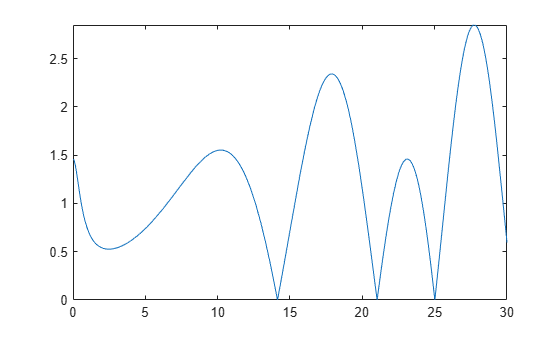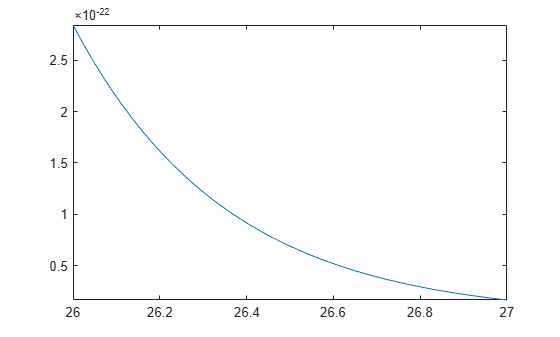## Recognize and Avoid Round-Off Errors

When approximating a value numerically, remember that floating-point results can be sensitive to the precision used. Also, floating-point results are prone to round-off errors. The following approaches can help you recognize and avoid incorrect results.

### Use Symbolic Computations When Possible

Performing computations symbolically is recommended because exact symbolic computations are not prone to round-off errors. For example, standard mathematical constants have their own symbolic representations in Symbolic Math Toolbox™:

```pi sym(pi)```
```ans = 3.1416 ans = pi```

Avoid unnecessary use of numeric approximations. A floating-point number approximates a constant; it is not the constant itself. Using this approximation, you can get incorrect results. For example, the `heaviside` special function returns different results for the sine of `sym(pi)` and the sine of the numeric approximation of `pi`:

```heaviside(sin(sym(pi))) heaviside(sin(pi))```
```ans = 1/2 ans = 1```

### Perform Calculations with Increased Precision

The Riemann hypothesis states that all nontrivial zeros of the Riemann Zeta function ζ(z) have the same real part ℜ(z) = 1/2. To locate possible zeros of the Zeta function, plot its absolute value |ζ(1/2 + iy)|. The following plot shows the first three nontrivial roots of the Zeta function |ζ(1/2 + iy)|.

```syms y fplot(abs(zeta(1/2 + i*y)), [0 30])```Use the numeric solver `vpasolve` to approximate the first three zeros of this Zeta function:

```vpasolve(zeta(1/2 + i*y), y, 15) vpasolve(zeta(1/2 + i*y), y, 20) vpasolve(zeta(1/2 + i*y), y, 25)```
```ans = 14.134725141734693790457251983562 ans = 21.022039638771554992628479593897 ans = 25.010857580145688763213790992563```

Now, consider the same function, but slightly increase the real part, $\zeta \left(\frac{1000000001}{2000000000}+iy\right)$. According to the Riemann hypothesis, this function does not have a zero for any real value y. If you use `vpasolve` with the 10 significant decimal digits, the solver finds the following (nonexisting) zero of the Zeta function:

```old = digits; digits(10) vpasolve(zeta(1000000001/2000000000 + i*y), y, 15)```
```ans = 14.13472514```

Increasing the number of digits shows that the result is incorrect. The Zeta function $\zeta \left(\frac{1000000001}{2000000000}+iy\right)$ does not have a zero for any real value 14 < y < 15:

```digits(15) vpasolve(zeta(1000000001/2000000000 + i*y), y, 15) digits(old)```
```ans = 14.1347251417347 + 0.000000000499989207306345i```

For further computations, restore the default number of digits:

`digits(old)`

### Compare Symbolic and Numeric Results

Bessel functions with half-integer indices return exact symbolic expressions. Approximating these expressions by floating-point numbers can produce very unstable results. For example, the exact symbolic expression for the following Bessel function is:

`B = besselj(53/2, sym(pi))`
```B = (351*2^(1/2)*(119409675/pi^4 - 20300/pi^2 - 315241542000/pi^6... + 445475704038750/pi^8 - 366812794263762000/pi^10 +... 182947881139051297500/pi^12 - 55720697512636766610000/pi^14... + 10174148683695239020903125/pi^16 - 1060253389142977540073062500/pi^18... + 57306695683177936040949028125/pi^20 - 1331871030107060331702688875000/pi^22... + 8490677816932509614604641578125/pi^24 + 1))/pi^2```

Use `vpa` to approximate this expression with the 10-digit accuracy:

`vpa(B, 10)`
```ans = -2854.225191```

Now, call the Bessel function with the floating-point parameter. Significant difference between these two approximations indicates that one or both results are incorrect:

`besselj(53/2, pi)`
```ans = 6.9001e-23```

Increase the numeric working precision to obtain a more accurate approximation for `B`:

`vpa(B, 50)`
```ans = 0.000000000000000000000069001456069172842068862232841396473796597233761161```

### Plot the Function or Expression

Plotting the results can help you recognize incorrect approximations. For example, the numeric approximation of the following Bessel function returns:

```B = besselj(53/2, sym(pi)); vpa(B, 10)```
```ans = -2854.225191```

Plot this Bessel function for the values of `x` around `53/2`. The function plot shows that the approximation is incorrect:

```syms x fplot(besselj(x, sym(pi)), [26 27])```